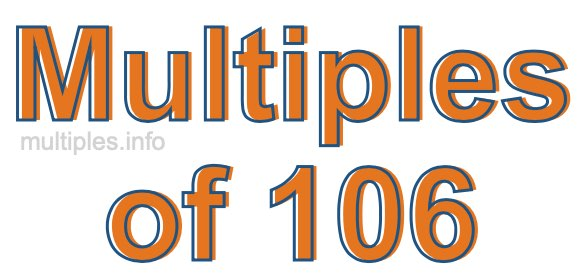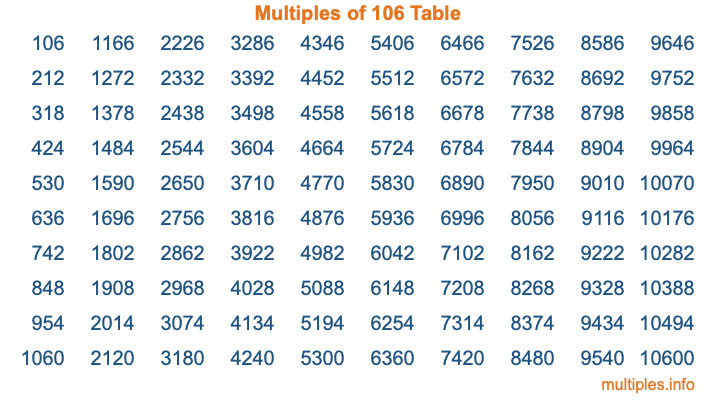Multiples of 106Welcome to the Multiples of 106 page. Here we will first teach you everything you will ever need to know about the multiples of 106, and then give you a study guide summary of everything we taught you to make sure you remember it all. Use this page to look up facts and learn information about the multiples of 106. This page will make you a multiples of one hundred six expert!

Definition of Multiples of 106
Multiples of 106 are all the numbers that when divided by 106 equal an integer. Each of the multiples of 106 are called a multiple. A multiple of 106 is created by multiplying 106 by an integer.

Therefore, to create a list of multiples of 106, you start with 1 multiplied by 106, then 2 multiplied by 106, then 3 multiplied by 106, and so on for as long as you want. Thus, the list of the first five multiples of 106 is 106, 212, 318, 424, and 530. To see a larger list of multiples of 106, see the printable image of Multiples of 106 further down on this page. We also have a category where you can choose any nth multiple of 106.

Multiples of 106 Checker
The Multiples of 106 Checker below checks to see if any number of your choice is a multiple of 106. In other words, it checks to see if there is any number (integer) that when multiplied by 106 will equal your number. To do that, we divide your number by 106. If the the quotient is an integer, then your number is a multiple of 106.

Is  a multiple of 106?

Least Common Multiple of 106 and ...
A Least Common Multiple (LCM) is the lowest multiple that two or more numbers have in common. This is also called the smallest common multiple or lowest common multiple and is useful to know when you are adding our subtracting fractions. Enter one or more numbers below (106 is already entered) to find the LCM.

Check out our LCM Calculator if you need more details about the Least Common Multiple or if you need the LCM for different numbers for adding and subtraction fractions.

nth Multiple of 106
As we stated above, 106 is the first multiple of 106, 212 is the second multiple of 106, 318 is the third multiple of 106, and so on. Enter a number below to find the nth multiple of 106.

th multiple of 106

Multiples of 106 vs Factors of 106
106 is a multiple of 106 and a factor of 106, but that is where the similarities end. All postive multiples of 106 are 106 or greater than 106. All positive factors of 106 are 106 or less than 106.

Below is the beginning list of multiples of 106 and the factors of 106 so you can compare:

Multiples of 106: 106, 212, 318, 424, 530, etc.

Factors of 106: 1, 2, 53, 106

As you can see, the multiples of 106 are all the numbers that you can divide by 106 to get a whole number. The factors of 106, on the other hand, are all the whole numbers that you can multiply by another whole number to get 106.

It's also interesting to note that if a number (x) is a factor of 106, then 106 will also be a multiple of that number (x).

Multiples of 106 vs Divisors of 106
The divisors of 106 are all the integers that 106 can be divided by evenly. Below is a list of the divisors of 106.

Divisors of 106: 1, 2, 53, 106

The interesting thing to note here is that if you take any multiple of 106 and divide it by a divisor of 106, you will see that the quotient is an integer.

Multiples of 106 Table
Below is an image of the first 100 multiples of 106 in a table. The table is in chronological order, column by column. The first column has the first ten multiples of 106, the second column has the next ten multiples of 106, and so on.The Multiples of 106 Table is also referred to as the 106 Times Table or Times Table of 106. You are welcome to print out our table for your studies.

Negative Multiples of 106
Although not often discussed or needed in math, it is worth mentioning that you can make a list of negative multiples of 106 by multiplying 106 by -1, then by -2, then by -3, and so on, to get the following list of negative multiples of 106:

-106, -212, -318, -424, -530, etc.

Multiples of 106 Summary
Below is a summary of important Multiples of 106 facts that we have discussed on this page. To retain the knowledge on this page, we recommend that you read through the summary and explain to yourself or a study partner why they hold true.

There are an infinite number of multiples of 106.

A multiple of 106 divided by 106 will equal a whole number.

106 divided by a factor of 106 equals a divisor of 106.

The nth multiple of 106 is n times 106.

The largest factor of 106 is equal to the first positive multiple of 106.

106 is a multiple of every factor of 106.

106 is a multiple of 106.

A multiple of 106 divided by a divisor of 106 equals an integer.

106 divided by a divisor of 106 equals a factor of 106.

Any integer times 106 will equal a multiple of 106.

Multiples of a Number
Here you can get the multiples of another number, all with the same attention to detail as we did for multiples of 106 on this page.

Multiples of
Multiples of 107
Did you find our page about multiples of one hundred six educational? Do you want more knowledge? Check out the multiples of the next number on our list!